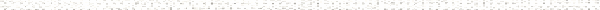An Engineering Statics Outline

 a. CouplesObjective: Recognize that at least two forces are necessary to cause only a rotation of a body and to utilize the concept of a couple and its symbol to represent combinations of forces that produce zero net force (only a tendency to rotate)Objective: Determine the moment of the couple from the forces producing it or balancing it, and recognize that a set of forces that can be represented as couples produce the same net moment and rotational effect about all points regardless of where they are applied.Objective: Recognize circumstances in which a multi-force interaction between two bodies can be represented as a couple, and determine the moment of the couple from what the couple interaction is balancing. b.Objective: Distinguish between fully equivalent loadings and statically equivalent loadingsObjective: Replace a general set of forces and couples with a single force at a location that needs to be determinedObjective: Recognize equivalence between forces acting along the same line of action <Objective: Recognize equivalence between several forces acting at a point and a single force at that point equal to their vector sum - full equivalenceObjective: Recognize equivalence between different combinations of forces that produce zero net force and the same total moment - equivalent couples <Objective: Replace a single force acting at a point with a statically equivalent force at a different point and an appropriate couple for moving a force perpendicular to its line of actionObjective: Replace a single force acting at a point with a statically equivalent force at a different point and an appropriate couple for moving a force to an arbitrary locationObjective: Replace two parallel forces acting in the same direction and sense with a single forceObjective: Replace two parallel forces acting in the same direction and opposite senses with a statically equivalent load (force and couple)Objective: Replace a general set of forces and couples with a force and couple at a given point c.Objective: Use symmetry to reduce three-dimensional situations to two-dimensional representationsObjective: Replace symmetrically distributed load per volume/area by a distributed load per lengthObjective: Replace a simple known distributed load per volume/ area/ length by a single force with an appropriate line of actionObjective: Represent unknown distributed forces due to contact between symmetrical flat bodies by a statically equivalent force acting at an unknown location somewhere in the contact areaObjective: Recognize the role of symmetry planes in determining the location of the center of gravityObjective: Determine the location of the center of gravity for bodies that can be decomposed into rectangular prismsObjective: Use tabulated results to determine the location of the center of gravity for bodies that can be decomposed into simple shapes (and recognize that the location of the center of gravity for prismatic bodies can be determined by integration). d. Representing Engineering ConnectionsObjective: Recognize two most common connections: fixed and pinnedObjective: Represent interactions between bodies joined by fixed connectionsObjective: Represent interactions between a cylindrical rod and contacting surfaces common in connectionsObjective: Recognize pin connections that can be modeled in 2-D even though forces act in different planes in 3-D: (a) symmetrical arrangements under symmetrical or planar loadings, (b) thin connected bodies under planar loadingsObjective: Represent interaction between bodies connected by a shared pin (roles of Newton's 3rd law and equilibrium)Objective: Represent interactions between bodies connected by link with two pinsObjective: Represent interactions between bodies connected by rollers, rockers, and pins in slotObjective: Represent interaction between bodies connected by a rigid sliding jointObjective: Represent interactions between bodies connected by a cable and a massless pulley